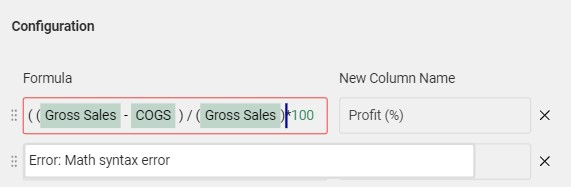# Formula NodeFormula node offers greater flexibility to transform your data. Packed with loads of features, you could type in the formula box to highlight fields and apply math operations including simple arithmetic, trigonometric functions and more.

• • How Do I Add The Formula Node?
• • What Are The Basics?
• • What Mathematical Operations Are Available?
• • How Do I Add, Re-Order, and Delete Formulas?
• • FAQ

## How Do I Add The Formula Node?

Add a Formula to any node in your Dataflow. Select any node and use the Transform (+) button open the list of options to select Formula. Note that you could work with the node in the dataflow tab or you could use the Properties panel.

## What Are The Basics?

### Simple Language for Writing Expressions

To make items distinguishable we have the following color coding:

1. Data fields - highlighted in green
2. Constants - green font
3. Functions - blue font

In the example below, BuiltInParameters.Volume is a data field, 215 is a constant value  and sin() and cos() are functions.

For a list of data fields, simply start typing the name of a header in your data and you will see a list of available options. When you have found the field you need in your expression press Enter to add it in your formula.

The same steps can be used to get a list of functions - simply type in the formula box to search and press enter to select.

## What Mathematical Operations Are Available?

Formula node supports most basic math operations, such as:

#### Arithmetic Operators

2. subtract (-)
3. multiply (*)
4. divide (/)

#### Trigonometric Functions

1. sin() - sine
2. asin() - arc sine
3. sinh() - hyperbolic sine
4. cos() - cosine
5. acos() - arc cosine
6. cosh() - hyperbolic cosine
7. tan()- tangent
8. atan() - arc tangent
9. tanh() - hyperbolic tangent

#### More Functions

1. log() - logarithm
2. log10() - log to base 10
3. pow(a,n) raise a to the power of n
4. rand() - return a random number
5. randint(a,b) - return random integers between values a and b

#### Constants

1. PI() = π = 3.14159265359

## How Do I Add, Re-Order, and Delete Formulas?

The Add Formula button allows you to add as many formulas as you wish. You can use the drag bar on the left to re-order formulas upwards or downwards to a desired position.

Each formula you write will create a new column to the right of your data. You could also delete a formula by clicking on the "x" button to the right of the formula.

## FAQ

#### 1. How do I know my expression makes sense?

There are a few errors warnings that will guide you in writing a complete expression. You can identify and error when the formula box is highlighted in red. For example:

Here are some common error warnings:

1. Equation missing: You will notice a red box around the formula field and the message at the bottom.
2. Math syntax error: There is a missing operator or bracket "(" in the formula.

#### 2. How come I cannot see the values from my formula?

In order to see the values, you will need to name the new column using the text field on the right of the formula box.